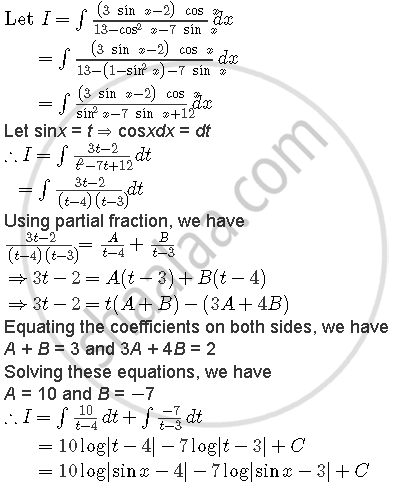Share

# Find Integral((3 Sin X - 2) Cos X)/(By3 - Cos^2 X- 7 Sin X) Dx - CBSE (Science) Class 12 - Mathematics

ConceptIntegration Using Trigonometric Identities

#### Question

Find int((3 sin x - 2) cos x)/(13 - cos^2 x- 7 sin x) dx

#### SolutionIs there an error in this question or solution?

#### Video TutorialsVIEW ALL 

Solution Find Integral((3 Sin X - 2) Cos X)/(By3 - Cos^2 X- 7 Sin X) Dx Concept: Integration Using Trigonometric Identities.
S